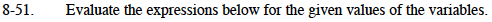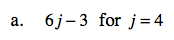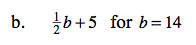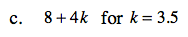### Home > MC1 > Chapter 8 > Lesson 8.2.3 > Problem8-51

8-51.For the expression below, you can substitute the values given for each variable and then simplify the expression.
It is important to remember that 2p really means two p's or (2)(p).
2p + 8 for p = 4
2(4) + 8 = 8 + 8 = 16
And, remember to follow the order of operations!21If the variable is substituted here, the expression becomes

$\frac{1}{2}(14) + 5.$

can you simplify it now?

128 + 4 (3.5)